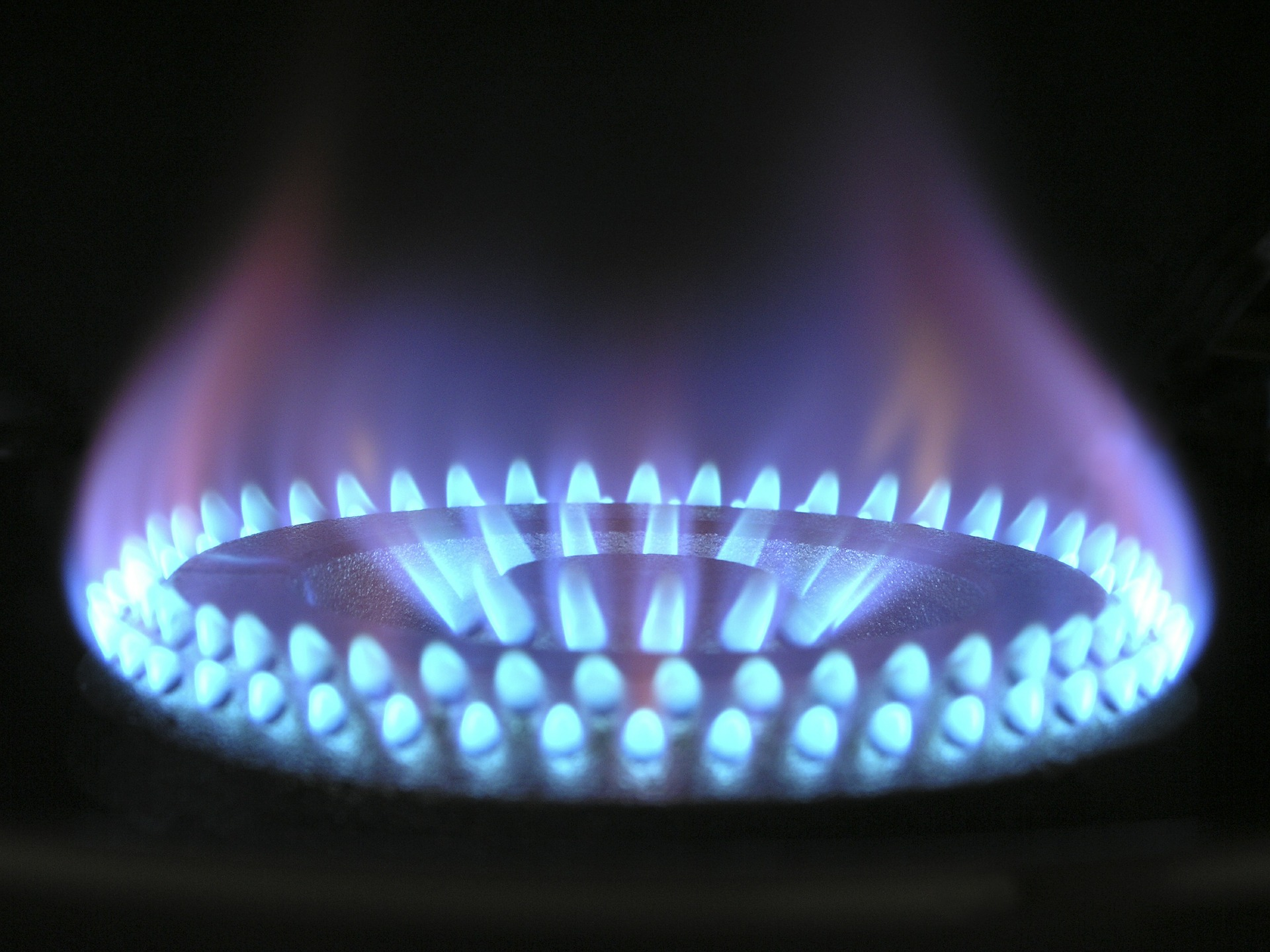# Conversion Higher Heating Value (HHV) to Lower Heating Value (LHV)

As a process engineer dealing with gas project, I usually involve in these terms, higher heating value (HHV) and lower heating value (LHV). Do you know the differences between these two?

In simple words, higher heating value (HHV) includes energy used to vaporize water. While lower heating value (LHV) excludes energy used to vaporize water.

Which water?

Water contained in the original energy form or created during the combustion process.

If we put them into equation, then it will be like this.

We get HHV by measuring from bomb calorimeter if the sample is oven dried (remember your basic chemistry experiment? 😊). While LHV is calculated from HHV.

So, how do we calculate LHV?

Based on publication by US Environmental Protection Agency, the relationship between HHV and LHV is:

LHV = HHV – 10.55 (W + 9H)

Where:

LHV        = lower heating value of fuel (BTU/lb)

HHV       = higher heating value of fuel (Btu/lb)

W            = weight percent of moisture in fuel

H             = weight percent of hydrogen in fuel

For fast analysis, I found that we can convert HHV to LHV using simple relationship below:

LHV = HHV / 1.11